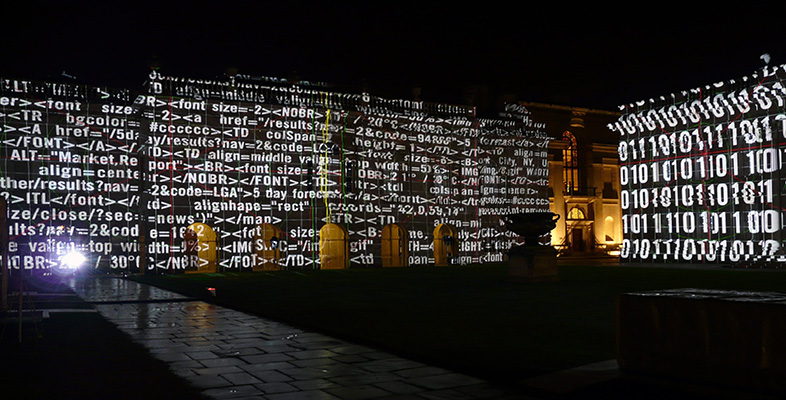Using numbers and handling data

Start this free course now. Just create an account and sign in. Enrol and complete the course for a free statement of participation or digital badge if available.

Free course

# 1.6 Multiplication and division by factors of ten

## 1.6.1 Getting comfortable with factors of ten

Moving a decimal point by one place changes the value of the number by a factor of ten. For instance, to multiply a value by ten you can just move the decimal point one place to the right: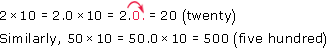Notice that if the starting number doesn't have a decimal point shown we can place an imaginary decimal point after the last digit, and a zero to the right of this, in order to help us see the change in the order of magnitude.

To multiply a number by 100, move the decimal point 2 places to the right: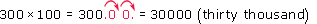The same principle applies to dividing by 10, 100, 1000 etc., except you move the decimal place to the left instead, thus making the original number smaller. For instance: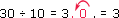Look at the animated examples in the second 'Info' box of your practice questions [Tip: hold Ctrl and click a link to open it in a new tab. (Hide tip)] . These animations illustrate how sequentially moving a decimal point by one place at a time changes that number by a factor of ten each time the point is moved.

In Table 2, each number in the second column differs from the one in the row immediately above or below it by a factor of ten, or in other words by one order of magnitude.

### Table 2 Names, units and symbols for factors of ten found within the decimal system of numbering

Name Number Order of magnitude Power Unit Symbol
Million1 000 0006106 mega M
Hundred thousand100 0005105
Ten thousand10 0004104
Thousand1 0003103 kilo k
Hundred1002102hectoh
Ten101101
One10100
Tenth0.1−110−1decid
Hundredth0.01−210−2centic
Thousandth0.001−310−3 milli m
Ten thousandth0.000 1−410−4
Hundred thousandth0.000 01−510−5
Millionth0.000 001−610−6 micro μ
Ten millionth0.000 000 1−710−7
Hundred millionth0.000 000 01−810−8
Billionth0.000 000 001−910−9nanon
The units shown in bold are those that you are likely to encounter on a daily basis in your work.

In the column marked 'Power', there is a 10 and a small, raised (superscript) number next to it for each of the names of the factors of ten mentioned. This superscript number is called the power or the exponent, and it indicates how many times the first number or 'base' (in this case a 10) is multiplied by itself in order to give the actual amount being signified. For instance, 102 ('ten squared'), means 10 × 10 = 100. Similarly, 104 means 10 × 10 × 10 × 10 = 10 000.

Notice also that numbers less than zero can be shown by a negative exponent. For instance, 10−1 means '1 divided by 10', where 1 ÷ 10 = 0.1. Similarly, 10−4 means '1 divided by 104', which is 1 ÷ 10 000, or 0.000 1. Note that any number to the power zero means that it is divided by itself and is therefore 1 (e.g. 10 ÷ 10 = 1), which explains why Table 2 states that 100 = 1.

An exponent will often have a number and a multiplication sign written before it, for example 2.4 × 103, which also means 2.4 × 1000, or 2400. An easy way to work out the value of numbers expressed as exponents is to move the decimal point the same number of places as the exponent.

• If the exponent is positive, then move the decimal point to the right by the same number of places as the exponent.

• If the exponent is negative, then move the decimal point to the left by the same number of places as the exponent.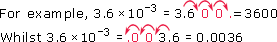S110_1